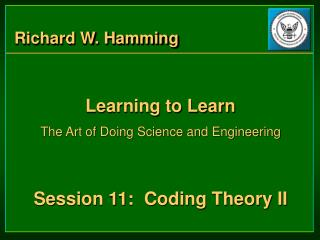DownloadDownload PresentationRichard W. Hamming

# Richard W. Hamming

Download Presentation## Richard W. Hamming

- - - - - - - - - - - - - - - - - - - - - - - - - - - E N D - - - - - - - - - - - - - - - - - - - - - - - - - - -
##### Presentation Transcript

1. Richard W. Hamming • Learning to Learn • The Art of Doing Science and Engineering • Session 11: Coding Theory II

2. Coding Theory II Overview • Two types of Coding • Source Encoding • Channel Encoding • How do we find the best source encoding? • Huffman encoding • How do we encode for a noisy channel? • Error correcting codes

3. The Best Source Encoding • We know efficient codes are related to probabilities. • Intuition leads to the solution: Prove this by exchanging any two lengths and the average code length decreases.

4. Deriving Huffman Encoding • Create the best encoding of symbols from their probabilities. • Build encoding tree from bottom up. • Merge 2 least frequent nodes, combine their probabilities, repeat. • Assign 0,1 to final 2 symbols. • Back down the tree, split symbols, assigning 0 or 1 appended to parent’s code.

5. Deriving Huffman Encoding Tree (Visual) p(s1)=0.4 0 p(s2)=0.2 10 1 p(s3)=0.2 110 11 p(s4)=0.1 p(s4 & s5 & s3)=0.4 1110 111 p(s4 & s5)=0.2 Combine probabilities, left to right Assign codes, right to left. p(s5)=0.1 1111

6. Optimality • Huffman trees give optimal (most efficient) codes. • Do we necessarily want optimal? • What characteristics do we want? • Efficiency (average code length)? • Efficiency and low variability? • Start from an optimum and move one way or the other. • Sometimes you can get a large change a desired characteristic but pay little in performance.

7. Why Huffman? • Write a program to do it. • Sending Huffman tree and encoded info saves channel or storage capacity. • Best possible data compression. • If anything can be gained by compression. • With uniform distribution, thus block code, no compression possible.

8. Channel Encoding • What to do with noise? • Error detection • Extra parity bit catches only 1 error. • Probability of 2 errors in a message of length n increases with n. • In a longer message parity bit takes up less channel capacity. • In a longer message 2nd error more likely. • Depends on probability p of error in a single bit.

9. Errors • Probability of 2,3,4, and higher errors depends mostly on np product. • np near 1 makes errors highly likely. • Very small np makes double, triple errors highly unlikely. • Tradeoff between error and efficiency. • Engineering judgment that requires a concrete case and a real n and p.

10. Error Handling • What if an error occurs? • Repeat the message • On marginal errors repeat messages will be clear • On systematic errors you will repeat error until you get a double error. Not a good strategy. • Strategy depends on the nature of the errors you expect.

11. Detecting Human Error • Encoding alphanumeric symbols. • Humans make different types of errors than machines • Changing one digit: 667 to 677 • Exchanging digits: 67 to 76 • Parity can’t cope with the two digit exchange error

12. xk is the value for the kth symbol of the message, 0 ≤ xk ≤ 36 Detecting Human Errors • Weighted code • Add 1 parity symbol to a message of length n-1 so the message checksum below is 0.

13. Different Types of Codes • ISBN is a weighted code with modulo 11. • Even/odd parity bit is for white noise • Weighted code is for human noise. • But machine can easily check code for mistake • Many types of codes not talked about. • Know what the noise is to determine type of code. • Design a code to meet the cope with your particular type of noise problem.

14. Creativity in Deriving Codes • Learn principles, then solve your problem. • Next lecture will tell how Hamming derived his codes. • If you were in the same situation Huffman or Hamming were in, could you come up with the codes if you had to? • Creativity is simple if you are prepared.

15. Parting Thoughts • Many scientists wasted the second half of their lives elaborating a famous theory. • Leave elaborations to others. • Follows from Hamming’s earlier statement, that those who come up with great ideas often don’t understand their own ideas as well as do those who follow. • Set great work aside, try to do something else.